# TExES Math 4-8: Linear Equations Chapter Exam

Exam Instructions:

Choose your answers to the questions and click 'Next' to see the next set of questions. You can skip questions if you would like and come back to them later with the yellow "Go To First Skipped Question" button. When you have completed the practice exam, a green submit button will appear. Click it to see your results. Good luck!

### Page 1

#### Question 3 3. Which one of the following equations could work for the following graph?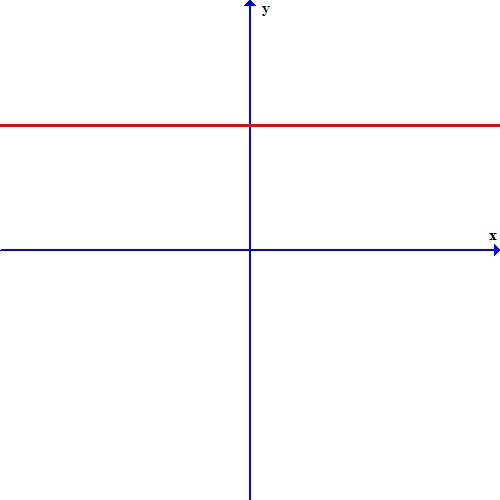### Page 2

#### Question 9 9. Which linear equation is shown below?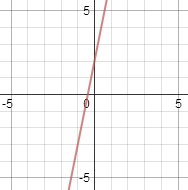### Page 4

#### Question 16 16. Based on this graph, what is the solution to the system of equations?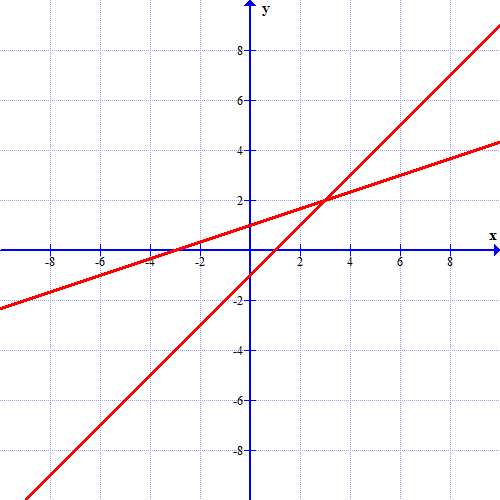#### Question 17 17. Which of these equations represents a line that is perpendicular to the line in the graph below?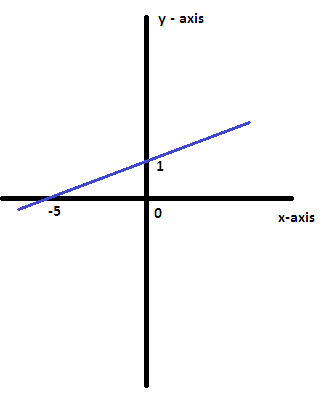#### Question 19 19. Which of the following is a solution to the system of equations shown in the graph below?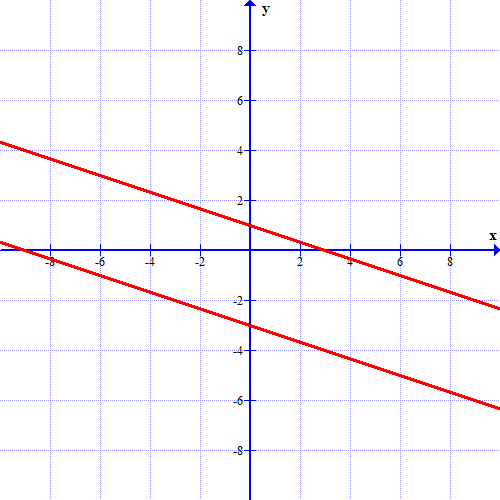### Page 5

#### Question 21 21. If the graph below has the equation Ax+By=C, which statement MUST be true?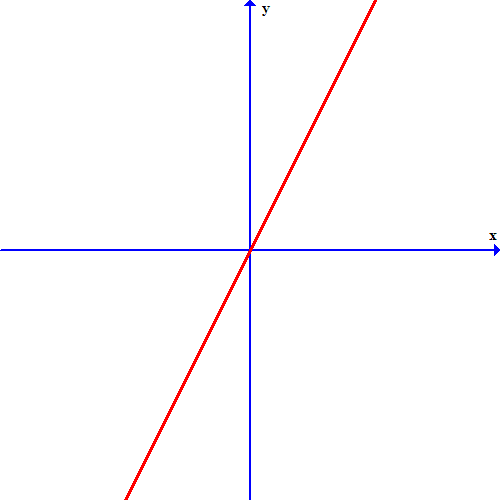### Page 6

#### TExES Math 4-8: Linear Equations Chapter Exam Instructions

Choose your answers to the questions and click 'Next' to see the next set of questions. You can skip questions if you would like and come back to them later with the yellow "Go To First Skipped Question" button. When you have completed the practice exam, a green submit button will appear. Click it to see your results. Good luck!

Support